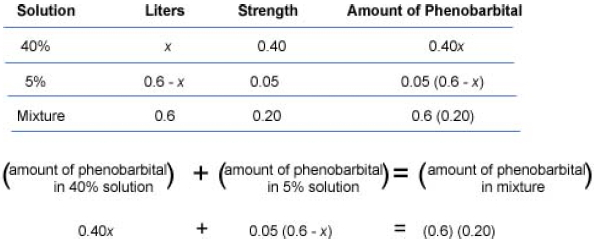Mixture Problems

Solving Mixture Problems

Any problem in which two or more quantities are combined to produce a different quantity, or a single quantity is separated into different quantities may be considered a mixture problem.

One type of mixture problem involves mixing liquids or solutions and evaluating the content or strength of the mixture. The following formulas are useful for evaluating these type of mixture problems:

amount of substance in the solution = quantity of solution x strength of solution (in percent)

In addition, the following formula is often used:

(amount of substance in mixture 1) + (amount of substance in mixture 2) = amount of substance in mixture

Example

Nathaniel works as a medical researcher. He has two solutions of phenobarbital, one at 40% strength and the other at 5% strength. How much of each solution must he mix to make 0.6 liters of a 20% phenobarbital solution?

Understand
Mix the 40% solution and the 5% solution to achieve 0.6 liter of a 20% solution. The amount of phenobarbital in a given solution is found by multiplying the number of liters by the percent strength.

Translate
Let x = number of liters of the 40% solution
Let 0.6 - x = number of liters of the 5% solutionSolve
0.40x + 0.05(0.6 - x) = (0.6)(0.20)
0.40x + 0.03 - 0.05x = 0.12                  distributive property on left; multiply on right
0.35x + 0.03 = 0.12                              combine like terms
0.35x = 0.09                                         subtract 0.03 from both sides
x = 0.26                                                divide both sides by 0.35